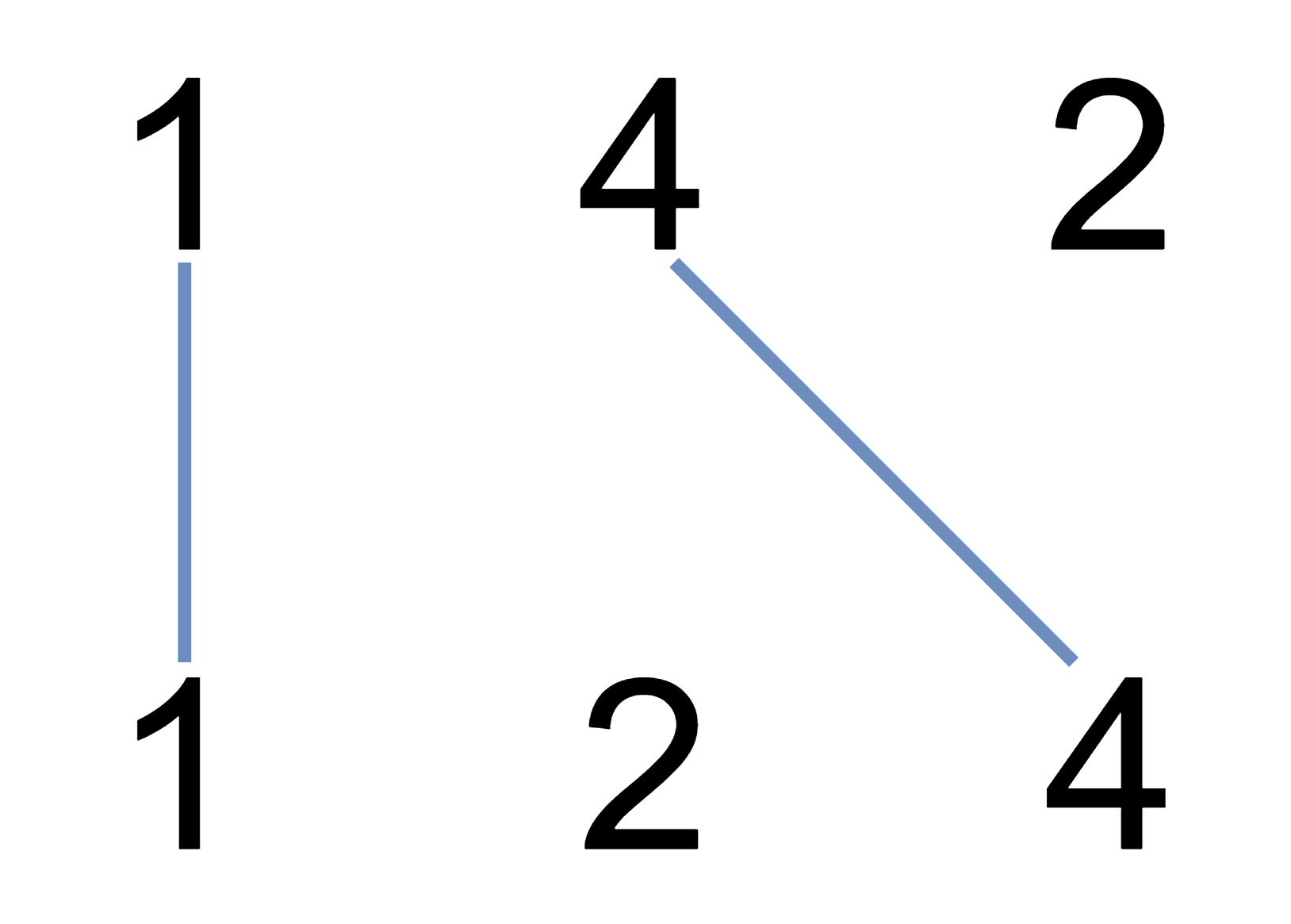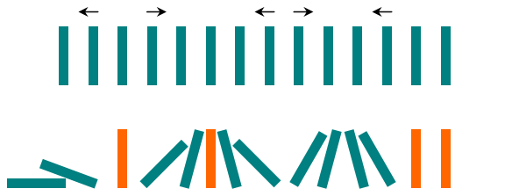Given a string `text`, we are allowed to swap two of the characters in the string. Find the length of the longest substring with repeated characters.

Example 1:

Example 2:

Example 3:

Example 4:

Example 5:

Constraints:

• `1 <= text.length <= 20000`
• `text` consist of lowercase English characters only

You have `d` dice, and each die has `f` faces numbered `1, 2, ..., f`.

Return the number of possible ways (out of `fd` total ways) modulo 10^9 + 7 to roll the dice so the sum of the face up numbers equals `target`.

Example 1:

Example 2:

Example 3:

Example 4:

Example 5:

Constraints:

• `1 <= d, f <= 30`
• `1 <= target <= 1000`

I just added unit tests and am planning to add functional test scripts to one of my project. What comes to your mind when you work on a project every day, already have had test scripts and there is a Jenkins server available? Right, automate it!

My compay uses BitBucket server. However, although there are several BitBucket server plugins available, all of them are not free (and they are even not cheap!). So far, I just want to invoke a Jenkins job when some events happen. Should we pay thousands of dollars for such a easy (relatively) use case? So I decide to find some free alternatives.

We write the integers of `A` and `B` (in the order they are given) on two separate horizontal lines.

Now, we may draw a straight line connecting two numbers `A[i]` and `B[j]` as long as `A[i] == B[j]`, and the line we draw does not intersect any other connecting (non-horizontal) line.

Return the maximum number of connecting lines we can draw in this way.

Example 1:Example 2:

Example 3:

Note:

1. `1 <= A.length <= 500`
2. `1 <= B.length <= 500`
3. `1 <= A[i], B[i] <= 2000`

Given a 2-dimensional `grid` of integers, each value in the grid represents the color of the grid square at that location.

Two squares belong to the same connected component if and only if they have the same color and are next to each other in any of the 4 directions.

The border of a connected component is all the squares in the connected component that are either 4-directionally adjacent to a square not in the component, or on the boundary of the grid (the first or last row or column).

Given a square at location `(r0, c0)` in the grid and a `color`, color the border of the connected component of that square with the given `color`, and return the final `grid`.

Example 1:

Example 2:

Example 3:

Note:

1. `1 <= grid.length <= 50`
2. `1 <= grid.length <= 50`
3. `1 <= grid[i][j] <= 1000`
4. `0 <= r0 < grid.length`
5. `0 <= c0 < grid.length`
6. `1 <= color <= 1000`

Three stones are on a number line at positions `a`, `b`, and `c`.

Each turn, let’s say the stones are currently at positions `x, y, z` with `x < y < z`. You pick up the stone at either position `x` or position `z`, and move that stone to an integer position `k`, with `x < k < z` and `k != y`.

The game ends when you cannot make any more moves, ie. the stones are in consecutive positions.

When the game ends, what is the minimum and maximum number of moves that you could have made? Return the answer as an length 2 array: `answer = [minimum_moves, maximum_moves]`

Example 1:

Example 2:

Note:

1. `1 <= a <= 100`
2. `1 <= b <= 100`
3. `1 <= c <= 100`
4. `a != b, b != c, c != a`

Two strings `X` and `Y` are similar if we can swap two letters (in different positions) of `X`, so that it equals `Y`.

For example, `"tars"` and `"rats"` are similar (swapping at positions `0` and `2`), and `"rats"` and `"arts"` are similar, but `"star"` is not similar to `"tars"`, `"rats"`, or `"arts"`.

Together, these form two connected groups by similarity: `{"tars", "rats", "arts"}` and `{"star"}`. Notice that `"tars"` and `"arts"`are in the same group even though they are not similar. Formally, each group is such that a word is in the group if and only if it is similar to at least one other word in the group.

We are given a list `A` of unique strings. Every string in `A` is an anagram of every other string in `A`. How many groups are there?

Example 1:

Note:

1. `A.length <= 2000`
2. `A[i].length <= 1000`
3. `A.length * A[i].length <= 20000`
4. All words in `A` consist of lowercase letters only.
5. All words in `A` have the same length and are anagrams of each other.
6. The judging time limit has been increased for this question.

There are `N` dominoes in a line, and we place each domino vertically upright.

In the beginning, we simultaneously push some of the dominoes either to the left or to the right.After each second, each domino that is falling to the left pushes the adjacent domino on the left.

Similarly, the dominoes falling to the right push their adjacent dominoes standing on the right.

When a vertical domino has dominoes falling on it from both sides, it stays still due to the balance of the forces.

For the purposes of this question, we will consider that a falling domino expends no additional force to a falling or already fallen domino.

Given a string “S” representing the initial state. `S[i] = 'L'`, if the i-th domino has been pushed to the left; `S[i] = 'R'`, if the i-th domino has been pushed to the right; `S[i] = '.'`, if the `i`-th domino has not been pushed.

Return a string representing the final state.

Example 1:

Example 2:

Note:

1. `0 <= N <= 10^5`
2. String `dominoes` contains only `'L`', `'R'` and `'.'`

Alice plays the following game, loosely based on the card game “21”.

Alice starts with `0` points, and draws numbers while she has less than `K` points. During each draw, she gains an integer number of points randomly from the range `[1, W]`, where `W` is an integer. Each draw is independent and the outcomes have equal probabilities.

Alice stops drawing numbers when she gets `K` or more points. What is the probability that she has `N` or less points?

Example 1:

Example 2:

Example 3:

Note:

1. `0 <= K <= N <= 10000`
2. `1 <= W <= 10000`
3. Answers will be accepted as correct if they are within `10^-5` of the correct answer.
4. The judging time limit has been reduced for this question.